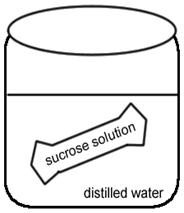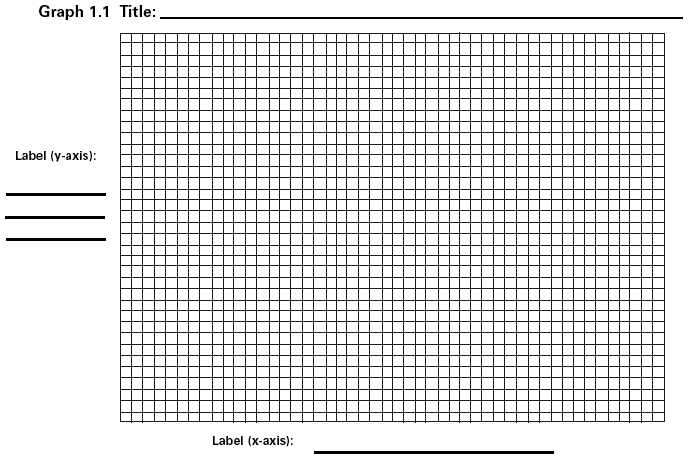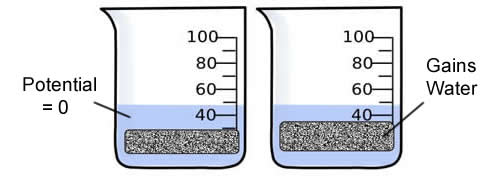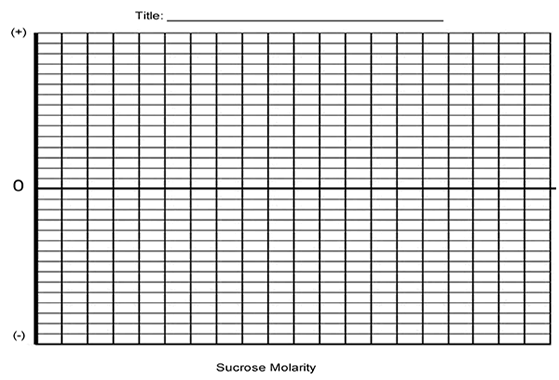Name:_____________________________________________________

Investigation: Osmosis and Water PotentialIn this lab, you will observe the process of osmosis and diffusion. You will also learn how to calculate water potential. If you are not familiar with these concepts, make sure that you have looked them up in your textbook. If you don't know what these terms mean, this lab is not going to make sense to you.

OBJECTIVES

• Investigate the processes of osmosis in a model of a membrane system
• Investigate the effect of solute concentration on water potential as it relates to living plant tissues

Exercise 1 - Osmosis Across a Membrane

1. Obtain 6 strips of dialysis tubing and tie an knot in one end of each.
2. Pour approximately 15-20 ml of each of the following solutions into separate bags.

 Distilled Water 0.4 M sucrose 0.8 M sucrose 0.2 M sucrose 0.6 M sucrose 1.0 M sucrose

3. Remove most of the air from the bag (but leave a little bit of space) and tie the baggie.
4. Blot the bags to remove any sugar that may have spilled, check the bags for leaks.
5. Record the mass of each baggie in the data table.
6. Fill six beakers with enough distilled water to cover your bags. Place a bag in each one (keep track of which bag is in which beaker)
7. Let the bag sit for 20-30 minutes.   -------- While this is running, set up potatoes for exercise 3.

Predict what you think will happen during the experiment. (Think about which bags will lose water and which will gain water.)

8. After 20-30 minutes, remove the baggies from the water, and carefully blot dry and record the final weight.

9. To calculate: percent change in mass= (final mass-initial mass)/ initial mass. Then multiply answer by 100.

 Contents in Bag Initial Mass Final Mass Mass Difference Time in Beaker % Change in Mass Distilled Water 0.2 M 0.4 M 0.6 M 0.8 M 1.0 M

11. Graph the results for your individual data that shows the relationship between %change in mass and the molarity of the solution. The independent variable is on the X axis, and the dependent variable is on the Y axisANALYSIS

1. Describe the relationship between the change in mass and the molarity of the sucrose in the dialysis tube.

Based on scientific principles, did you observe what you expected? If not, suggest a reason or possible errors in set-up or data gathering.

2. Why did you calculate the percent change in mass rather than simply using the change in mass?

3. Predict what would happen to the mass of each bag in this experiment if all the bags were placed in 0.4 M sucrose solution instead of distilled water. Explain your response.

4. A dialysis bag is filled with distilled water and then placed in a sucrose solution. The bag's initial mass is 20 g, and its final mass is 18 g. Calculate the percent change of mass, showing your calculations.

EXERCISE 2 - Determining the Water Potential of Potato Cells

In animal cells, the movement of water into and out of the cell is influenced by the relative concentration of solute on either side of the cell membrane. If water moves out of the cell, the cell will shrink. If water moves into the cell, the cell may swell or even burst. In plant cells, the presence of a cell wall prevents the cells from bursting, but pressure does eventually build up inside the cell and affects the process of osmosis. When the pressure inside the cell becomes large enough, no additional water will accumulate in the cell even. So movement of water through the plant tissue cannot be predicted simply through knowing the relative solute concentrations on either side of the plant cell wall. Instead, the concept of water potential is used to predict the direction in which water will diffuse through living plant tissues.

In a general sense, the water potential is the tendency of water to diffuse from one area to another. Water potential is expressed in in bars, a metric unit of pressure equal to about 1 atmosphere and measured with a barometer.

Consider a potato cell is placed in pure water. Initially the water potential outside the cell is 0 and is higher than the water potential inside the cell. Under these conditions there will be a net movement of water into the cell. The pressure potential inside the cell will increase until the cell reaches a state of equilibrium.Directions:

1. Pour 100 mL of your assigned solution (it will be one of the six solutions listed above in Exercise 2) into a beaker. Slice a potato into 4 equal cylinders or slices, they will resemble french fries.
2. Determine the mass of all 4 potato cylinders together and record.
3. Place the cylinders into the beaker with your assigned solution and cover with plastic wrap. Leave overnight.
4. Remove the cylinders from the beakers and record the mass. Determine the temperature of the room. ________
5. Complete the table and graph your results.

 Contents in Bag Initial Mass Final Mass Mass Difference %Change in Mass Distilled Water 0.2 M 0.4 M 0.6 M 0.8 M 1.0 M6. Determine the molar concentration of the potato cores. This would be the sucrose molarity in which the mass of the potato core does not change. To find this, draw the straight line on your graph that best fits your data. The point at which this line crosses the x axis represents the molar concentration of sucrose with a water potential that is equal to the potato tissue water potential.

What is the Molar concentration of the cores? ___________

7. Calculate the solute potential ( Ψ ) for the sucrose solution using the formula below. Show your work!

Solute Potential Formula:        Ψ = -iCRT

i = ionization constant (for sucrose, this is 1 because sucrose does not ionize in water)
C = molar sucrose concentration at equilibrium (determined from graph)
R = pressure constant (0.0831 liter bar/mole °K ) | T = temperature °K (273 + °C )

8. Explain water potential and describe how it affects osmosis.

9. Explain how you would determine the molarity of a potato.

Extension: Design an Experiment to Test an Unknown

You are given a solution of sucrose that has an unknown molarity (.2, .4,.6, .8, 1.0), how could you use potatoes, distilled water, or other known solutions to determine the molarity of your unknown? - Be clear in your design, use another page and staple to this one. Conduct your experiment and include the results with your determination of which solution you had. Solutions will be color-coded by the instructor. Complete the CER chart below.

Claim:

Evidence:

Reasoning:

Suggest ways to improve your experimental design or to obtain greater confidence in your claim. If you were given another day to work on this, what would you do?